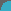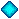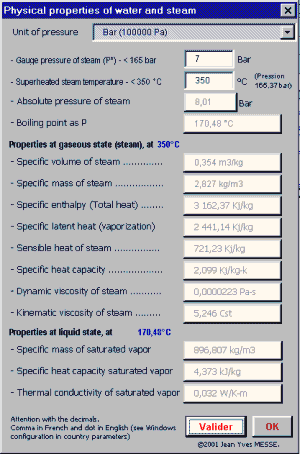Functions of calculation written in VBA for the calculation of the properties of water and the steam. Function of calculation of pressure loss on the steam pipes.
French site
| Home | Thematic | Tables | Programs | Library | Download | Links | Contact | Forum |_You are in the heading :--

ThermoVapor program (Function)
 Programs DevExcel DevExcel ? Estimate calculation Estimate (next) Synthesis, Graphic Library Help PsychroSI Psychrometric calculation Swimming pool Mixed air calculation AeroDuct Duct sizing Pressure loss coefficients Fan HydroTherm HydroWater Pipes sizing ThermExcel Pipes sizing HydroWater Local pressure loss Control valve Pump Orifice plate Expansion ThermoVapor Pipes sizing Local pressure loss Control valve Orifice plate Functions AeroGaz ThermGaz Aerogaz program Thermgaz program Local pressure loss Control valve Orifice plate Sundry programs AutoPlanning TriFileLexicon employed for the steam (see thematic: Distribution of steam)

The module of integrated calculation makes it possible to establish all the physical characteristics of water, of vapor and of the vapor overheated

 Steam in a saturated state(1) Steam in an overheated state(2)The calculation of the physical characteristics of the saturated steam (1) can be done from the relative pressure or conversely according to the temperature of the steam or the two parameters in the case of use of the overheated steam (2)

Functions of calculation written in VBA

There is a great number of functions integrated available immediately in Excel. The personalized functions written in VBA for the ThermoVapeur program can be used like the functions integrated of Excel with the proviso that of installed the ThermoVapor program in Excel.

The functions below are used in the sorter and can be re-used on other worksheets.

The functions below are used in the working file and can be re-used on other worksheets.

Formulas of computation of pressure loss on the piping.

Functions of calculation for the ThermoVapor program

Calculation of flow of water
- P_therm = Thermal power (Watts)
- Delta_T = Variation in temperature enters the inlet and the outlet (°K)
- Mas_V = density (kg/m3)
- CM = Specific heat: in kJ/(kg K)
Function = Debit(P_therm, Delta_T, Cm, Mas_V)

Calculation of the Reynolds number according to the dynamic viscosity of the fluid
- Visc_dyn = Dynamic viscosity, value E-6. kg/(m S)
- Vit = Speed (in m/s)
- Mas_V = density (in kg/m3)
- Dia (Internal diameter pipe) (in mm)
Function = Reynolds(Mas_Vol, Vit, Dia, Visc)

Calculation of the Reynolds number according to cinematic viscosity
- Vit = Speed (in m/s)
- Visc = Viscosity in centistoke
- Dia = Internal diameter pipe in mm
Function = Reynolds1(Vit, Visc, Dia)

Calculation of the factor of friction according to the equation of Colebrook (iterative Method)
- Rugo = Roughness (in mm)
- DI = Diameter line (in mm)
- Re = Reynolds
Function = Friction1(Rugo, Re, Di)

Speed of water in m/s
- Deb = Volumic flow of vapor in m3/h
- Dia = Diameter (mm)
Function = Vites(Deb, Dia)

Calculation of the dynamic Pressure
- Dens = density in kg/m3
- Vit = Speed (in m/s)
Function = Pdyn(Dens, Vit)

Linear pressure loss
- Friction (Coefficient of pressure loss)
- Density (in kg/m3)
- Diam_tube (Internal diameter pipe) (in mm)
- Speed (in m/s)
Function = Pdc(Friction, Diam_tube, Density, Speed, Nature)

Correction of expansion
- PdcTot = Total linear pressure loss
- Pres = Relative pressure relating at tle inlet of the pipe
Function = Function Expan(PdcTot, Pres)

Calculation of the module of pressure loss ( Coefficients K depend on the diameter used)
- Ke = Module of pressure loss
- Index = Factor of the module of pressure loss
- Dia = Diameter line (in mm)
Function = Module(Ke, Dia, Index)

Functions for calculations of the properties of water and the steam

See thematic: Vapor tables

Kinematic viscosity
- T = Temperature (in °C)
- Mas_V = density (in kg/m3)
- Visc_dyn = Dynamic viscosity, value E-6. kg/(m S)
Function = Visc_cine(T, Mas_V)

Dynamic viscosity of water, value E-6. kg/(m S)
Range of validity: Up to 500 °C and 600 bar
- T = Temperature (in °C)
- V = Volume in m3/kg
Function = Visc_dyn(T, V)

Density of the saturated steam in kg/m3
Range of validity: Up to 300 bar
- P = relative Pressure in Bar
Function = MassVol(P)

Density of the overheated steam in kg/m3
Range of validity: Up to 350 °C and 300 bar
- T = Temperature (in °C)
- P = relative Pressure in Bar
Function = Mass_vol(T, P)

Latent heat of the saturated steam in kJ / kg K
Range of validity: Up to 300 bar
- P = relative Pressure in Bar
Function = Chlatente(P)

Latent heat of the overheated steam in kJ / kg K
Range of validity: Up to 350 °C and 300 bar
- T = Temperature (in °C)
- P = Relative pressure of the steam in Bar
Function = Chlatent(T, P)

Specific enthalpy of the saturated steam (total heat) in kJ / kg K
Range of validity: Up to 300 bar
- P = Relative pressure in Bar
Function = Enthalp(P)

Specific enthalpy of the overheated steam (total heat) in kJ / kg K
Range of validity: Up to 350 °C and 300 bar
- T = Temperature (in °C)
- P = Relative pressure in Bar
Function = Enthal(T, P)

Enthalpy of ebullient water in kJ / kg K
Range of validity: Up to 300 bar
- P = relative Pressure in Bar
Function = ChH2O(P)

Enthalpy of the water overheated in kJ / kg K
Range of validity: Up to 350 °C and 300 bar
- T = Temperature (in °C)
- P = relative Pressure Bar
Function = ChH2O1(T, P)

Specific heat of the steam in kJ / kg K
Range of validity: Up to 300 bar
- P = relative Pressure in Bar
Function = ChMas(P)

Pressure of vaporization in absolute bar
Range of validity: Up to 350 °C
- T = Temperature (in °C)
Function = Pression(T)

Temperature of vaporization
Range of validity: Up to 300 bar
- P = relative Pressure in Bar
Function = TempVap(P)

Various functions

Calculation of the diaphragm (in mm) according to Standard NFX 10-101
- Diam_int = Internal diameter of the pipe, mm
- Flow = Water flow, l/h
- Pdc = Pressure loss to be created, Bar
- Temp = Fluid temperature, °C
Function = D_diaphr(Diam_int, Flow, Pdc, Temp, P)

Calculation flow of vapor according the Kv
- Qm = Mass flow rate of vapor, kg/h
- P1 = Relative pressure of the vapor - upstream, Bar
- P2 = Relative pressure of the vapor - downstream, Bar
- Temp = Temperature of the overheated vapor, °C
Function = Débit_Kv(Kv, P1, P2, Temp)

Calculation Kv-value of the valve for the vapor
- Qm = Mass flow rate of the vapor, kg/h
- P1 = Relative pressure of the vapor - upstream, Bar
- P2 = Relative pressure of the vapor - downstream, Bar
- Temp = temperature of the overheated vapor, °C
Function = Module_Kv(Qm, P1, P2, Temp)

Conversion pdc into modules
- Speed = Actual speed of circulation, m/s
- Temp = Temperature of water, °C
- Pdc = Pressure loss to be created, Pa
- P = Relative pressure of the vapor in Bar
Function = Module_Pdc1(Pdc, Speed, Temp, P)

Last update: